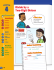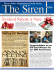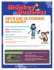# D I V I S I O N ...

## Transcription

D I V I S I O N ...
```5.5
5.5
Division of Polynomials
(5-33)
289
DIVISION OF POLYNOMIALS
We began our study of polynomials in Section 5.3 by learning how to add, subtract,
and multiply polynomials. In this section we will study division of polynomials.
In this
section
●
Dividing a Polynomial by a
Monomial
●
Dividing a Polynomial by a
Binomial
●
Synthetic Division
●
Division and Factoring
Dividing a Polynomial by a Monomial
You learned how to divide monomials in Section 5.1. For example,
6x 3
6x 3 (3x) 2x 2.
3x
We check by multiplying. Because 2x 2 3x 6x 3, this answer is correct. Recall
that a b c if and only if c b a. We call a the dividend, b the divisor, and
c the quotient. We may also refer to a b and a as quotients.
b
We can use the distributive property to find that
3x(2x 2 5x 4) 6x 3 15x 2 12x.
So if we divide 6x 3 15x 2 12x by the monomial 3x, we must get
2x 2 5x 4. We can perform this division by dividing 3x into each term of
6x 3 15x2 12x:
6x 3 15x 2 12x 6x 3 15x 2 12x
3x
3x
3x
3x
2
2x 5x 4
In this case the divisor is 3x, the dividend is 6x 3 15x 2 12x, and the quotient is
2x 2 5x 4.
E X A M P L E
1
hint
Recall that the order of operations gives multiplication and
division an equal ranking
and says to do them in order
from left to right. So without
parentheses,
12x 5 2x 3
actually means
12x 5 3
x .
2
Dividing polynomials
Find the quotient.
a) 12x 5 (2x 3)
b) (20x 6 8x 4 4x 2) (4x 2)
Solution
a) When dividing x 5 by x 3, we subtract the exponents:
12x 5
12x 5 (2x 3) 6x 2
2x 3
The quotient is 6x 2. Check:
6x 2 2x 3 12x 5
b) Divide each term of 20x 6 8x 4 4x 2 by 4x 2:
20x 6 8x 4 4x 2
20x 6 8x4 4x 2
2 2
2
4x
4x 2
4x
4x
4
2
5x 2x 1
4
2
The quotient is 5x 2x 1. Check:
4x 2(5x 4 2x 2 1) 20x 6 8x 4 4x 2
■
290
(5-34)
Chapter 5
Exponents and Polynomials
Dividing a Polynomial by a Binomial
We can multiply x 2 and x 5 to get
(x 2)(x 5) x 2 3x 10.
So if we divide x 2 3x 10 by the factor x 2, we should get the other factor
x 5. This division is not done like division by a monomial; it is done like long
division of whole numbers. We get the first term of the quotient by dividing the
first term of x 2 into the first term of x 2 3x 10. Divide x 2 by x to get x.
x
x 2 x2
x
3
0
1
x 2 2x
5x
x2 x x
Multiply: x(x 2) x 2 2x.
Subtract: 3x (2x) 5x.
Now bring down 10. We get the second term of the quotient (below) by dividing
the first term of x 2 into the first term of 5x 10. Divide 5x by x to get 5.
x5
x 2 x2
x
3
0
1
x 2 2x
5x 10
5x 10
0
5x x 5
Multiply: 5(x 2) 5x 10.
Subtract: 10 (10) 0.
So the quotient is x 5 and the remainder is 0. If the remainder is not 0, then
dividend (divisor)(quotient) (remainder).
If we divide each side of this equation by the divisor, we get
dividend
remainder
quotient .
divisor
divisor
When dividing polynomials, we must write the terms of the divisor and the dividend in descending order of the exponents. If any terms are missing, as in the next
example, we insert terms with a coefficient of 0 as placeholders. When dividing
polynomials, we stop the process when the degree of the remainder is smaller than
the degree of the divisor.
E X A M P L E
2
hint
Students usually have the
most difficulty with the subtraction part of long division.
So pay particular attention to
that step and double check
Dividing polynomials
Find the quotient and remainder for (3x 4 2 5x) (x 2 3x).
Solution
Rearrange 3x 4 2 5x as 3x 4 5x 2 and insert the terms 0x 3 and 0x 2:
3x 2 9x 27
2
x 4
x
030
x 2
2
x 3x3
5x
4
3
3x 9x
9x 3 0x 2 0x 3 (9x 3) 9x 3
9x 3 27x 2
27x 2 5x
27x 2 81x
76x 2
5.5
Division of Polynomials
(5-35)
291
The quotient is 3x 2 9x 27, and the remainder is 76x 2. Note that the degree
of the remainder is 1, and the degree of the divisor is 2. To check, verify that
■
(x 2 3x)(3x 2 9x 27) 76x 2 3x 4 5x 2.
E X A M P L E
3
Rewriting a ratio of two polynomials
x9
Write 4x
in the form
3
2x 3
remainder
quotient .
divisor
study
tip
Have you ever used excuses to
avoid studying? (“Before I can
study, I have to do my laundry
and go to the bank.”) Since the
average attention span for
20 minutes, it is better to take
breaks from studying to run
errands and to do laundry
than to get everything done
before you start studying.
Solution
Divide 4x 3 x 9 by 2x 3. Insert 0 x2 for the missing term.
2x 2 3x 4
x 3
x
02
9 4x 3 (2x) 2x 2
2x 34
x
3
2
4x 6x
6x 2 x
0x 2 (6x 2) 6x 2
2
6x 9x
8x 9 x (9x) 8x
8x 12
3 9 (12) 3
Since the quotient is 2x 2 3x 4 and the remainder is 3, we have
4x 3 x 9
3
2x 2 3x 4 .
2x 3
2x 3
To check the answer, we must verify that
(2x 3)(2x 2 3x 4) 3 4x 3 x 9.
■
Synthetic Division
When dividing a polynomial by a binomial of the form x c, we can use synthetic
division to speed up the process. For synthetic division we write only the essential
parts of ordinary division. For example, to divide x3 5x2 4x 3 by x 2,
we write only the coefficients of the dividend 1, 5, 4, and 3 in order of
descending exponents. From the divisor x 2 we use 2 and start with the following arrangement:
2
1 5 4 3
(1 x 3 5x 2 4x 3) (x 2)
Next we bring the first coefficient, 1, straight down:
2
1 5 4 3
↓ Bring down
1
We then multiply the 1 by the 2 from the divisor, place the answer under the 5, and
then add that column. Using 2 for x 2 allows us to add the column rather than
subtract as in ordinary division:
2
1 52 4 3
Multiply
1 3
292
(5-36)
Chapter 5
Exponents and Polynomials
We then repeat the multiply-and-add step for each of the remaining columns:
2
1 3 2 7
← Remainder
Multiply
1 52 64 3
4
Quotient
From the bottom row we can read the quotient and remainder. Since the degree of
the quotient is one less than the degree of the dividend, the quotient is 1x 2 3x 2.
The remainder is 7.
The strategy for getting the quotient Q(x) and remainder R by synthetic
division can be stated as follows.
Strategy for Using Synthetic Division
1.
2.
3.
4.
5.
6.
List the coefficients of the polynomial (the dividend).
Be sure to include zeros for any missing terms in the dividend.
For dividing by x c, place c to the left.
Bring the first coefficient down.
Multiply by c and add for each column.
Read Q(x) and R from the bottom row.
CAUTION
Synthetic division is used only for dividing a polynomial by
the binomial x c, where c is a constant. If the binomial is x 7, then c 7. For
the binomial x 7 we have x 7 x (7) and c 7.
E X A M P L E
4
Using synthetic division
Find the quotient and remainder when 2x 4 5x 2 6x 9 is divided by x 2.
Solution
Since x 2 x (2), we use 2 for the divisor. Because x 3 is missing in the
dividend, use a zero for the coefficient of x 3:
← 2x 4 0 x 3 5x 2 6x 9
2 2 0 5 6 9
4 8 6 0
Multiply
2 4 3 0 9
← Quotient and remainder
Because the degree of the dividend is 4, the degree of the quotient is 3. The quotient
is 2x 3 4x 2 3x, and the remainder is 9. We can also express the results of this
mainder
division in the form quotient re
:
divisor
2x 4 5x 2 6x 9
9
2x 3 4x 2 3x x2
x2
■
Division and Factoring
To factor a polynomial means to write it as a product of two or more simpler polynomials. If we divide two polynomials and get 0 remainder, then we can write
dividend (divisor)(quotient)
5.5
Division of Polynomials
(5-37)
293
and we have factored the dividend. The dividend factors as the divisor times the
quotient if and only if the remainder is 0. We can use division to help us discover
factors of polynomials. To use this idea, however, we must know a factor or a
possible factor to use as the divisor.
E X A M P L E
5
Using synthetic division to determine factors
Is x 1 a factor of 6x 3 5x 2 4x 3?
Solution
We can use synthetic division to divide 6x 3 5x 2 4x 3 by x 1:
1 6 5 4 3
↓
6
1 3
6 1 3 0
Because the remainder is 0, x 1 is a factor, and
6x 3 5x 2 4x 3 (x 1)(6x 2 x 3).
E X A M P L E
6
Using division to determine factors
Is a b a factor of a 3 b3?
Solution
Divide a 3 b 3 by a b. Insert zeros for the missing a2b- and ab 2-terms.
a2 ab b2
a ba
3
00
b3
3
2
a ab
a2b 0
a2b ab2
ab2 b3
ab2 b3
0
Because the remainder is 0, a b is a factor, and
a3 b3 (a b)(a2 ab b2).
WARM-UPS
■
1.
2.
3.
4.
5.
6.
7.
8.
9.
10.
If a b c, then c is the dividend. False
The quotient times the dividend plus the remainder equals the divisor. False
(x 2)(x 3) 1 x 2 5x 7 is true for any value of x. True
The quotient of (x 2 5x 7) (x 3) is x 2. True
If x 2 5x 7 is divided by x 2, the remainder is 1. True
To divide x 3 4x 1 by x 3, we use 3 in synthetic division. False
We can use synthetic division to divide x 3 4x 2 6 by x 2 5. False
If 3x 5 4x 2 3 is divided by x 2, the quotient has degree 4. True
If the remainder is zero, then the divisor is a factor of the dividend. True
If the remainder is zero, then the quotient is a factor of the dividend. True
■
294
5. 5
(5-38)
Chapter 5
Exponents and Polynomials
EXERCISES
answers to these questions. Use complete sentences.
1. What are the dividend, divisor, and quotient?
If a b c, then the divisor is b, the dividend is a, and
the quotient is c.
2. In what form should polynomials be written for long division?
For long division polynomials should be written with the
exponents in descending order.
3. What do you do about missing terms when dividing polynomials?
If the term x n is missing in the dividend, insert the term
0 x n for the missing term.
4. When do you stop the long division process for dividing
polynomials?
Stop the long division process when the remainder has a
smaller degree than the divisor.
5. What is synthetic division used for?
Synthetic division is used only for dividing by a binomial
of the form x c.
6. What is the relationship between division of polynomials
and factoring polynomials?
The remainder is zero if and only if the dividend is a factor
of the divisor.
Find the quotient. See Example 1.
7. 36x 7 (3x 3) 12x 4
8. 30x 3 (5x) 6x 2
9. 16x 2 (8x 2) 2
10. 22a3 (11a2) 2a
11. (6b 9) 3 2b 3
12. (8x 2 6x) 2 4x 2 3x
13. (3x 2 6x) (3x) x 2
14. (5x 3 10x 2 20x) (5x) x 2 2x 4
15. (10x 4 8x 3 6x 2) (2x 2) 5x 2 4x 3
16. (9x 3 6x 2 12x) (3x) 3x 2 2x 4
7
17. (7x 3 4x 2) (2x) x 2 2x
2
3
5
3
2
2
18. (6x 5x ) (4x ) x 2
4
Find the quotient and remainder as in Example 2. Check by
using the formula
dividend (divisor)(quotient) remainder.
19. (x 2 8x 13) (x 3) x 5, 2
20. (x 2 5x 7) (x 3) x 2, 1
21. (x 2 2x) (x 2) x 4, 8
22. (3x) (x 1) 3, 3
23. (x 3 8) (x 2) x 2 2x 4, 0
24. (y 3 1) (y 1) y 2 y 1, 0
25. (a3 4a 5) (a 2) a2 2a 8, 11
(w 3 w 2 3) (w 2) w 2 3w 6, 9
(x 3 x 2 x 3) (x 1) x 2 2x 3, 6
(a3 a2 a 4) (a 2) a2 3a 7, 18
(x 4 x x 3 1) (x 2) x 3 3x 2 6x 11, 21
(3x 4 6 x 2 3x) (x 2)
3x 3 6x 2 11x 19, 44
31. (5x 2 3x 4 x 2) (x 2 2) 3x 2 1, x 4
32. (x 4 2 x 3) (x 2 3) x 2 x 3, 3x 7
1
5 7
33. (x 2 4x 2) (2x 3) x , 2
4 4
1
7
2
34. (x 5x 1) (3x 6) x , 15
3
3
2
1 56
2
35. (2x x 6) (3x 2) x , 3
9 9
3
5 9
2
36. (3x 4x 1) (2x 1) x , 2
4 4
Write each expression in the form
remainder
quotient .
divisor
See Example 3.
2x
x
38. 37. x5
x1
10
1
2 1 x5
x1
x2
x2 9
39. 40. x1
x3
1
18
x 1 x 3 x1
x3
x3 1
x3
41. 42. x2
x2
8
7
2
x 2x 4 x 2 2x 4 x2
x2
x 3 2x
2x 2 3
43. 44. 2x
x2
2
3
x x x
2x
x 2 4x 9
x 2 5x 10
45. 46. x2
x3
21
16
x 6 x 2 x3
x2
3x 3 4x 2 7
2x 3 x 2 3
47. 48. x1
x2
6
17
3x 2 x 1 2x 2 5x 10 x1
x2
Use synthetic division to find the quotient and remainder when
the first polynomial is divided by the second. See Example 4.
26.
27.
28.
29.
30.
49. x 3
x2
50. x 3
x2
5x 2 6x 3,
3x, 3
6x 2 3x 5,
9x 24, 67
x2
x3
5.5
C(x) 0.03x 300x.
2
295
The average cost per bicycle is given by
C(x)
AC(x) .
x
a) Find a formula for AC(x). AC(x) 0.03x 300
b) Is AC(x) a constant function? No
c) Why does the average cost look constant in the accompanying figure?
Because AC(x) is very close to 300 for x less than 15,
the graph looks horizontal.
Cost (in thousands of dollars)
51. 2x 2 4x 5, x 1 2x 6, 11
52. 3x 2 7x 4, x 2 3x 13, 30
53. 3x 4 15x 2 7x 9, x 3
3x 3 9x 2 12x 43, 120
54. 2x 4 3x 2 5, x 2
2x 3 4x 2 5x 10, 25
55. x 5 1, x 1
x 4 x 3 x 2 x 1, 0
56. x6 1, x 1
x 5 x 4 x 3 x 2 x 1, 0
57. x 3 5x 6, x 2
x 2 2x 1, 8
58. x 3 3x 7, x 4
x 2 4x 13, 45
59. 2.3x 2 0.14x 0.6, x 0.32
2.3x 0.596, 0.79072
60. 1.6x 2 3.5x 4.7, x 1.8
1.6x 6.38, 16.184
For each pair of polynomials, determine whether the first polynomial is a factor of the second. Use synthetic division when
possible. See Examples 5 and 6.
61. x 4, x 3 x 2 11x 8 No
62. x 4, x 3 x 2 x 48 No
63. x 4, x 3 13x 12 Yes
64. x 1, x 3 3x 2 5x No
65. 2x 3, 2x 3 3x 2 4x 6 Yes
66. 3x 5, 6x 2 7x 6 No
67. 3w 1, 27w 3 1 Yes
68. 2w 3, 8w 3 27 Yes
69. a 5, a3 125 Yes
70. a 2, a6 64 Yes
71. x 2 2, x 4 3x 3 6x 4 Yes
72. x 2 3, x 4 2x 3 4x 2 6x 3 Yes
Factor each polynomial given that the binomial following each
polynomial is a factor of the polynomial.
73. x 2 6x 8, x 4 (x 4)(x 2)
74. x 2 3x 40, x 8 (x 8)(x 5)
75. w 3 27, w 3 (w 3)(w 2 3w 9)
76. w 3 125, w 5 (w 5)(w 2 5w 25)
77. x3 4x 2 6x 4, x 2 (x 2 2x 2)(x 2)
78. 2x 3 5x 7, x 1 (2x 2 2x 7)(x 1)
79. z 2 6z 9, z 3 (z 3)(z 3)
80. 4a2 20a 25, 2a 5 (2a 5)(2a 5)
81. 6y 2 5y 1, 2y 1 (2y 1)(3y 1)
82. 12y 2 y 6, 4y 3 (4y 3)(3y 2)
Solve each problem.
83. Average cost. The total cost in dollars for manufacturing x
professional racing bicycles in one week is given by the
polynomial function
(5-39)
Division of Polynomials
4
3
C(x)
2
1
AC(x)
0
0
5
10
Number of bicycles
15
FIGURE FOR EXERCISE 83
84. Average profit. The weekly profit in dollars for manufacturing x bicycles is given by the polynomial
P(x) 100x 2x 2. The average profit per bicycle is given
by AP(x) P(x)
.
x
Find AP(x). Find the average profit per
bicycle when 12 bicycles are manufactured.
AP(x) 100 2x, \$124
85. Area of a poster. The area of a rectangular poster advertising a Pearl Jam concert is x 2 1 square feet. If the length
is x 1 feet, then what is the width? x 1 feet
86. Volume of a box. The volume of a shipping crate is
h3 5h2 6h. If the height is h and the length is h 2,
then what is the width? h 3
h
?
h2
FIGURE FOR EXERCISE 86
87. Volume of a pyramid. Ancient Egyptian pyramid builders
knew that the volume of the truncated pyramid shown in
the figure on the next page is given by
H(a3 b3)
V ,
3(a b)
296
(5-40)
Chapter 5
Exponents and Polynomials
where a2 is the area of the square base, b 2 is the area of the
square top, and H is the distance from the base to the top.
Find the volume of a truncated pyramid that has a base of
900 square meters, a top of 400 square meters, and a height
H of 10 meters.
6,333.3 cubic meters
88. Egyptian pyramid formula. Rewrite the formula of the
previous exercise so that the denominator contains the
number 3 only.
H(a2 ab b2)
V 3
GET TING MORE INVOLVED
b
b
H
a
a
FIGURE FOR EXERCISE 87
5.6
In this
section
●
Factoring Out the Greatest
Common Factor (GCF)
●
Factoring Out the Opposite
of the GCF
●
Factoring the Difference of
Two Squares
●
Factoring Perfect Square
Trinomials
●
Factoring a Difference or
Sum of Two Cubes
●
Factoring a Polynomial
Completely
●
Factoring by Substitution
89. Discussion. On
a
test
a
student
divided
3x3 5x2 3x 7 by x 3 and got a quotient of
3x2 4x and remainder 9x 7. Verify that the divisor
times the quotient plus the remainder is equal to the dividend. Why was the student’s answer incorrect?
90. Exploration. Use synthetic division to find the quotient
when x 5 1 is divided by x 1 and the quotient when
x 6 1 is divided by x 1. Observe the pattern in the first
two quotients and then write the quotient for x 9 1
divided by x 1 without dividing.
FACTORING POLYNOMIALS
In Section 5.5 you learned that a polynomial could be factored by using division:
If we know one factor of a polynomial, then we can use it as a divisor to obtain the
other factor, the quotient. However, this technique is not very practical because the
division process can be somewhat tedious, and it is not easy to obtain a factor to
use as the divisor. In this section and the next two sections we will develop better
techniques for factoring polynomials. These techniques will be used for solving
equations and problems in the last section of this chapter.
Factoring Out the Greatest Common Factor (GCF)
A natural number larger than 1 that has no factors other than itself and 1 is called a
prime number. The numbers
2, 3, 5, 7, 11, 13, 17, 19, 23
are the first nine prime numbers. There are infinitely many prime numbers.
To factor a natural number completely means to write it as a product of prime
numbers. In factoring 12 we might write 12 4 3. However, 12 is not factored
completely as 4 3 because 4 is not a prime. To factor 12 completely, we write
12 2 2 3 (or 22 3).
We use the distributive property to multiply a monomial and a binomial:
6x(2x 1) 12x 2 6x
If we start with 12x 2 6x, we can use the distributive property to get
12x 2 6x 6x(2x 1).
We have factored out 6x, which is a common factor of 12x 2 and 6x. We could
have factored out just 3 to get
12x 2 6x 3(4x 2 2x),
but this would not be factoring out the greatest common factor. The greatest common factor (GCF) is a monomial that includes every number or variable that is a
factor of all of the terms of the polynomial.
```

### Session 130: Unpacking Multiplication and Division With Fractions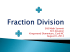### MINISTERUL EDUCAŢIEI AL REPUBLICII MOLDOVA Îţi dorim mult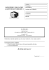### Managers` Towering Personality with 14Qs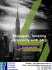### Divide Whole Numbers### Factoring Using the Distributive Property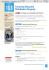### Malta International Airport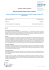### 8.4 Dividing Integers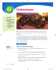### Divide by a Two-Digit Divisor - Macmillan/McGraw-Hill